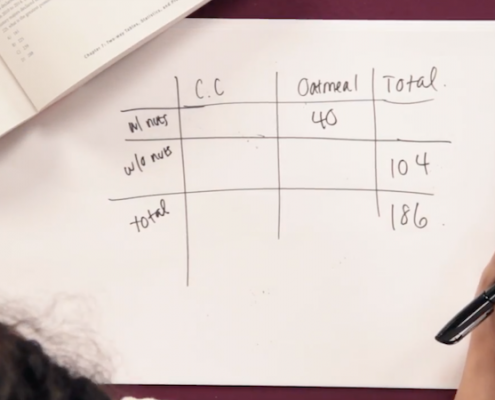# How to find average velocity

One of the SAT’s most challenging concepts is Average Rate, also called Average Speed. Often found in complex word problems, this type of question is one many students are less familiar with so don’t get nervous if you don’t know how to approach it yet!

Let’s review two important equations to remember and look at how this concept appears on the SAT!

The first important formula to memorize is: D = R x T. This stands for Distance = Rate x Time. I like to think of it as the “DIRT” formula and writing it this way is the easiest way for me to remember. It is perfectly acceptable to also think of it as Time = Distance / Rate or as Rate = Distance / Time. Usually the “Rate” is speed but it could be anything “per” anything. In a word problem, if you see the word “per” you know this is a question involving rates.

The second formula is: Average Rate = Total Distance / Total Time. This is its own special concept and you will notice that it is NOT an Average of the Speeds (which would be something like the Sum of the Speeds / the Number of Different Speeds or what we know as the Arithmetic Mean). Average Rate is completely different. Let’s look at an example question:

Let’s try another one that will require us to use both the “Average Rate” formula and the “DIRT” formula.

Marion spent all day on a sightseeing trip in Tuscany. First she boarded the bus which went 15mph through a 30 mile section of the countryside. The bus then stopped for lunch in Florence before continuing on a 3 hour tour of the city’s sights at speed of 10mph. Finally, the bus left the city and drove 40 miles straight back to the hotel. Marion arrived back at her hotel exactly 2 hours after leaving Florence. What was the bus’s average rate for the entire journey?

Related text  How to drop a pin

To find the “Average Rate” of the bus, we know we will need to find the Total Distance and the Total Time, so let’s see how we can use the D = R x T formula to find the missing info.

For the first part of the trip, we know that 30 miles = 15mph x T, so we know that T = 2 hours. For the middle part of the trip, we know that D = 10mph x 3 hours, so we know that D = 30 miles. For the last part of the trip, we know that 40 miles = R x 2 hours, so we know that R = 20mph.

Now we can find the Total Distance and the Total Time. Total Distance = 30 miles + 30 miles + 40miles = 100 miles. Total Time = 2 hours + 3 hours + 2 hours = 7 hours.

So the Average Rate = 100 miles/ 7 hours = 14.28mph.

Now that we’ve become pros at calculating Average Rates, let’s look at a more challenging question:

Tracey ran to the top of a steep hill at an average pace of 6 miles per hour. She took the exact same trail back down. To her relief, the descent was much faster; her average speed rose to 14 miles per hour. If the entire run took Tracey exactly one hour to complete and she did not make any stops, what is the length of trail in miles one way?

For the way up the hill, we know that D = 6mph x T.

For the way down the hill, we know that D = 14mph x T. Since we went know that the distance up the hill was the same as the distance down the hill, we can pick a number for D. Let’s choose “84” since it is a multiple of both 6 and 14. If 84 = 6mph x T, then we know that T = 14 hours. If 84 = 14mph x T, then we know that T = 6 hours.

Related text  How to make homemade wine

Now we can use another formula, the Average Rate formula, to find the average speed for the WHOLE trip.

Average Rate = Total Distance / Total Time

Using our Picked Number of 84, we know that the Total Distance traveled would be 168 miles. The Total Time is 14 hours + 6 hours = 20 hours. So the

Average Rate = 168 miles / 20 hours = 8.4 mph.

It doesn’t matter that Tracey didn’t “really” go 168 miles, or that we know she didn’t “really” go 20 hours. We Picked a Number just so that we could find the ratio of the Total Distance to the Total Time in order to calculate the Average Rate of the ENTIRE journey.

Now that we have found the Average Rate for the whole trip, we can plug it in to the “DIRT” formula to find the ACTUAL distance for the entire journey.D = R x T

D = 8.4mph x 1 hour

We know that T = 1 hour because the problem told us so. Therefore, the actual distance for the entire trip was 8.4 miles. The problem asks how many miles the trail was one way. 8.4 / 2 = 4.2. The answer to the question is 4.2 miles.

You could also solve this problem in other ways, including using a system of equations and substitution, but it’s nice to know that you can pick a number for the Distance traveled and use it to find the Average Rate for the whole journey. Be on the lookout for those trips where the distance there and back is the same!